Courses

# The Fractional Quantum Hall Effect (Part-1) Physics Notes | EduRev

## Physics : The Fractional Quantum Hall Effect (Part-1) Physics Notes | EduRev

The document The Fractional Quantum Hall Effect (Part-1) Physics Notes | EduRev is a part of the Physics Course Basic Physics for IIT JAM.
All you need of Physics at this link: Physics

The Fractional Quantum Hall Effect
We've come to a pretty good understanding of the integer quantum Hall effect and the reasons behind it's robustness. Indeed, some of the topological arguments in the previous chapter are so compelling that you might th
ink the Hall resistivity of an insulator has to be an integer. But each of these arguments has a subtle loophole and ultimately they hol
d only for non-interacting electrons. As we will now see, much more interesting things can happen when we include interactions.

As with the integer quantum Hall effect, these interesting things were first discovered by experimenters rather than theorists. Indeed, it came as a great surprise to the community when, in 1982, plateaux in the Hall resistiv
ity were seen at non-integer filling fractions. These plateaux were first seen at filling fraction v= 1/3 and 2/3 , and later at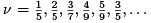in the lowest Landau level and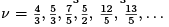in higher Landau leve
ls, as well as many others. There are now around 80 quantum Hall plateaux that have been observed. A number of these are shown below13: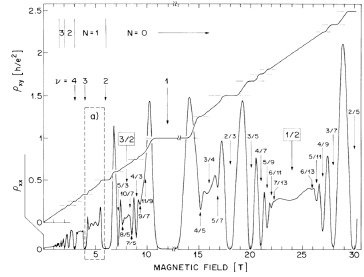There's two things that we can say immediately. First, the interactions between electrons must be playing some role. And second, the answer to why these plateaux form is likely to be very hard. Let's see why.

Suppose, for the sake of argument, that we have v < 1 so that the lowest Landau level is partially filled. Each Landau level can house N = AB =Φ0 (spin polarised)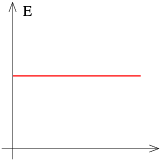Figure 25: Density of states in the lowest Landau level without interactions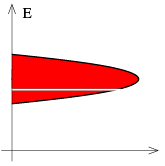Figure 26: ...and with interactions (with only a single gap at v = 1/3 shown.

electrons, where B is the magnetic field and A is the area of the sample. This is a macroscopic number of electrons. The number of ways to fill vN of these states is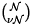which, using Stirling’s formula, is approximately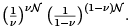This is a

ridiculously large number: an exponential of an exponential. The ground state of any partially filled Landau level is wildly, macroscopically degenerate.
Now consider the effect of the Coulomb interaction between electrons,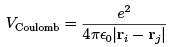(3.1)
On general grounds, we would expect that such an interaction would lift the degeneracy of ground states. But how to pick the right one? The approach we’re taught as undergraduates is to use perturbation theory. But, in this case, we’re stuck with extraordinarily degenerate perturbation theory where we need to diagonalise a macro-scopically large matrix. That’s very very hard. Even numerically, no one can do this for more than a dozen or so particles.
We can, however, use the experiments to intuit what must be going on. As we mentioned above, we expect the electron interactions to lift the degeneracy of the Landau level, resulting in a spectrum of states of width ~ ECoulomb. The data would be nicely explained if this spectrum had gaps at the filling fractions v where Hall states are seen. In the picture above, we’ve depicted just a single gap at v =1/3. Presumably though there are many gaps at different fractions: the more prominent the plateaux, the larger the gap.
Then we can just re-run the story we saw before: we include some disorder, which introduces localised states within the gap, which then gives rise both to the plateaux in pxy and the observed pxx = 0. The bigger the gap, the more prominent the observed plateaux. This whole story requires the hierarchy of energy scales,
ℏωB≫ECoulomb ≫ Vdisorder
We will assume in what follows that this is the case. The question that we will focus on instead is: what is the physics of these fractional quantum Hall states?
In what follows, we will take advantage of the difficulty of a direct theoretical attack on the problem to give us license to be more creative. As we’ll see, the level of rigour in the thinking about the fractional quantum Hall effect is somewhat lower than that of the integer effect. Instead, we will paint a compelling picture, using a number of different approaches, to describe what’s going on.

Laughlin States
The first approach to the fractional quantum Hall effect was due to Laughlin14, who described the physics at filling fractions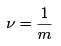with m an odd integer. As we’ve explained above, it’s too difficult to diagonalise the Hamiltonian exactly. Instead Laughlin did something very bold: he simply wrote down the answer. This was motivated by a combination of physical insight and guesswork. As we will see, his guess isn’t exactly right but, it’s very close to being right. More importantly, it captures all the relevant physics.

The Laughlin Wavefunction
Laughlin’s wavefunction didn’t come out of nowhere. To motivate it, let’s start by considering an illuminating toy model.

Two Particles
Consider two particles interacting in the lowest Landau level. We take an arbitrary central potential between them,
V = V (|r1 r2|)
Recall that in our rst courses on classical mechanics we solve problems like this by using the conservation of angular momentum. In quantum physics, this means that we work with eigenstates of angular momentum. As we sa
w in Section 1.4, if we want to talk about angular momentum in Landau levels, we should work in symmetric gauge. The single parti
cle wavefunctions in the lowest Landau level take the form (1.30)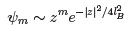with z = x—iy. These states are localised on a ring of radius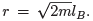The exponent m of these wavefunctions labels the angular momentum. This can be seen by acting with the angular momentum operator (1.31),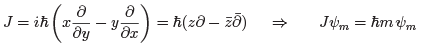Rather remarkably, this information is enough to solve our two-particle problem for any potential V! As long as we neglect mixing between Landau levels (which is valid if ℏwB ≫ V) then the two-particle eigenstates for any potential must take the form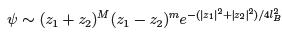where M, m are non-negative integers, with M determining the angular momentum of the centre of mass, and m the relative angu
lar momentum. Note that here, and below, we’ve made no attempt to normalise the wavefunctions.
It’s surprising that we can just write down eigenfunctions for a complicated potential V(r) without having to solve the Schro
dinger equation. It’s even more surprising that all potentials V(r) have the same energy eigenstates. It is our insistence that we lie in the lowest Landau level that allows us to do this.

Many-Particles
Unfortunately, it’s not possible to generalise arguments similar to those above to uniquely determine the eigenstates for N > 2 particles. Nonetheless, on general grounds, any lowest Landau level wavefunction must take the form,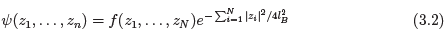for some analytic function f (z). Moreover, this function must be anti-symmetric under exchange of any two particle zi o zj, reflecting the fact that the underlying electrons are fermions.

Offer running on EduRev: Apply code STAYHOME200 to get INR 200 off on our premium plan EduRev Infinity!

,

,

,

,

,

,

,

,

,

,

,

,

,

,

,

,

,

,

,

,

,

;